Excellence in Broadband Dielectric Spectroscopy • Impedance Spectroscopy • Conductivity Spectroscopy

## Frequency Domain Measurement

The purpose is to measure the complex permittivity spectra ε*(ω) and conductivity spectra σ*(ω) of a sample material. The material may be solid or liquid and is usually placed between two electrodes which form a capacitor.Fig. 1: Principle of a frequency domain dielectric and conductivity measurement.

For compact formulas, complex notation is used and indicated by the asterisk.

From the sample capacitors impedance spectrum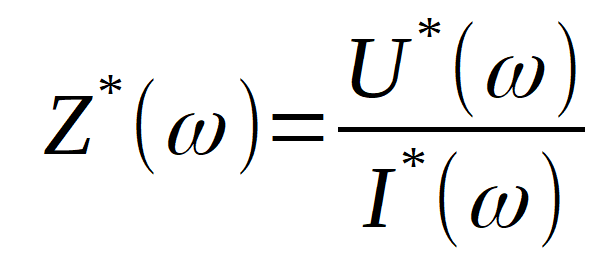the complex sample capacity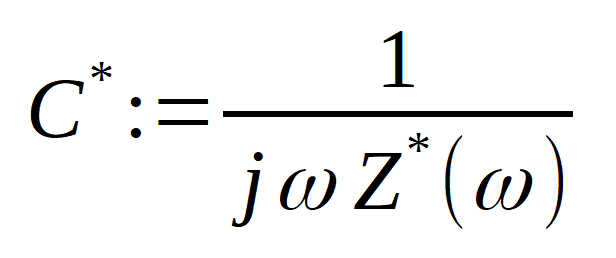is calculated. C*(ω) is directly related to the permittivity spectrum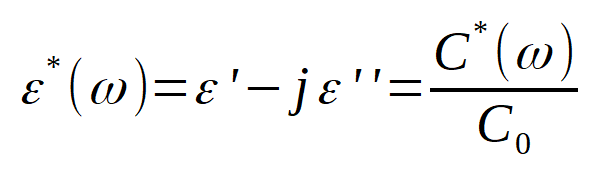where C0 is the capacity of the empty electrodes without sample material in between. If the electrodes are arranged parallel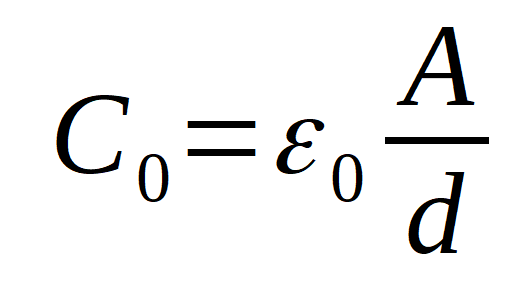where A denotes the area of one electrode, d the spacing between the electrodes and ε0=8.854*10-12 As/Vm is the vacuum permittivity. It should be noted that ε*(ω) generally includes a permittivity contribution from molecular dipoles and a conductivity contribution from free charge carriers. The same applies to the conductivity spectrum which is another representation of ε*(ω), i.e.,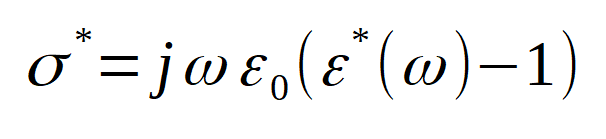The term −1 takes the charge contribution of the empty electrodes (vacuum permittivity)  into acount, resulting in ε*(ω) = 1 and σ*(ω) = 0 for the vacuum. Refer to Dielectric Spectroscopy and Impedance Spectroscopy of Materials for details and typical properties of ε*(ω) and σ*(ω).